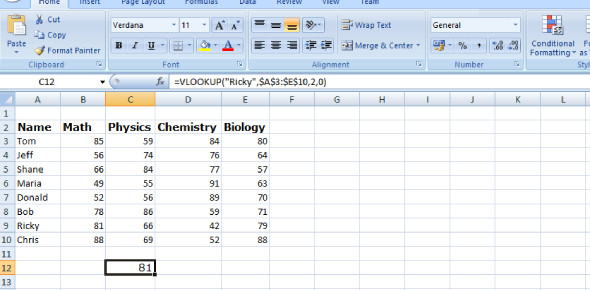# MCQ Quiz: Microsoft Excel Functions! Trivia

9 Questions | Total Attempts: 120SettingsDo you have a strong knowledge of Microsoft Excel functions? Microsoft Excel is said to be the most challenging Microsoft tool for people to understand because of its ability to handle different types of data. In this quiz, you will get to test out just how much you know the tool and its many features. Give it a shot and see if you might need a refresher course to polish up your skills when done.

Related Topics
• 1.
A function or formulae in Excel begins with a __
• A.

*

• B.

/

• C.

=

• 2.
_______ function is used to find the total of given values.
• A.

Average ()

• B.

Sum ()

• C.

Sumif ()

• 3.
To know the total number of records can be found by ______ function.
• A.

Count ()

• B.

Sum ()

• C.

Total ()

• 4.
Excel automatically updates the calculated values if Formula and Functions are used.
• A.

True

• B.

False

• 5.
Which of the following will give the average of data from B1 to B5 ?
• A.

= Average (B1:B5)

• B.

= (B1+B2+B3+B4+B5) / 5

• C.

= Average ( B1:B5 / 5)

• 6.
A student wrote =Sum(B1B5) to find the total. ______ is missing from the function?
• A.

-

• B.

;

• C.

:

• 7.
We have to enter the formula or function for each cell. It cannot be copied.
• A.

True

• B.

False

• 8.
A row is indicated by a number and a column by a letter.
• A.

True

• B.

False

• 9.
We can display the formula or function used in a cell instead of values.
• A.

True

• B.

False